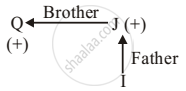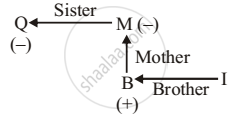# Which of the Following Means 'I is the Nephew of Q'?(I) Q% J = I (Ii) Q/M × B% I (Iii) Q/I = B% Q - Logical Reasoning

MCQ

Read the following information carefully to choose best option for the question.

• 'M% L' means that M is brother of L.
• 'L × M' means that L is mother of M.
• 'L/ M' means that L is sister of M.
• 'L = M' means that L is father of M.

Which of the following means 'I is the nephew of Q'?

(i) Q% J = I

(ii) "Q"/"M" × "B"% "I"

(iii) "Q"/"I" = "B" % "Q"

#### Options

• Only iii

• Only i

• Only ii

• None of these

#### Solution

Only ii

Explanation:

From (i),

Q % J = IGender of I cannot be determined.

From ii,

"Q"/"M" xx "B" % "I"So, from the above diagram, I is the nephew of Q.

Concept: Blood Relationships (Entrance Exam)
Is there an error in this question or solution?